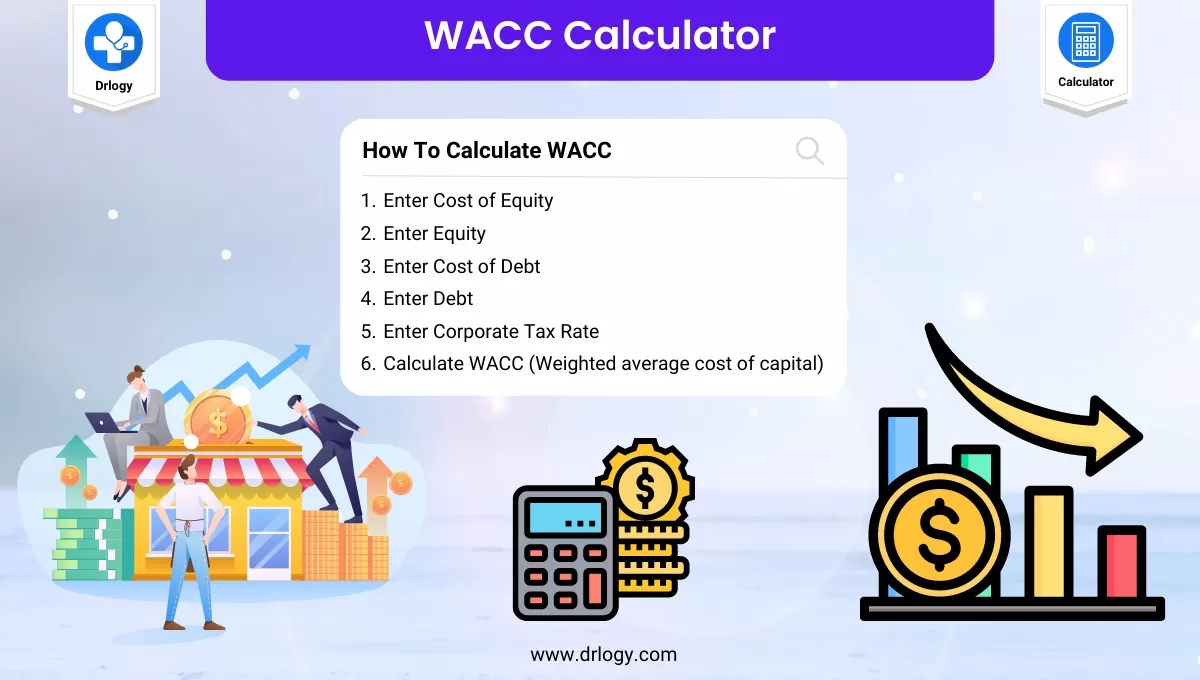• Health
• Pet
• Unit Conversion
• Medical# WACC Calculator (Weighted Average Cost of Capital) For Business

## WACC Calculator

###### Result

The weighted average cost of capital (WACC) is the rate that a company is expected to pay on average to all its security holders to finance its assets.

## WACC Calculator Overview

• WACC calculator is a financial tool used to calculate the overall cost of a company's capital.
• It takes into account the cost of debt and the cost of equity, and calculates the weighted average of the two.
• WACC is used as a discount rate to evaluate investment projects.
• It is an essential tool for financial analysts, investors, and managers to determine the profitability and value of an investment.
• The calculation of WACC is based on several factors such as the company's capital structure, tax rate, and market conditions.
• WACC can also be used to determine the minimum rate of return that a company needs to achieve to satisfy its investors.
• Regular use of WACC calculator can help companies make informed financial decisions and maximize shareholder value.

## What is WACC

• WACC is a financial metric that calculates the overall cost of a company's capital.
• It represents the average cost of funds that a company has raised from both debt and equity sources.
• WACC takes into account the relative proportions of debt and equity in a company's capital structure, as well as the cost of each source of financing.
• The cost of debt is calculated by taking into account the interest rate and tax benefits associated with it.
• The cost of equity is calculated by taking into account the expected return demanded by equity investors.
• WACC is used as a discount rate in discounted cash flow (DCF) analysis to determine the present value of future cash flows.
• WACC is an important tool for companies to evaluate potential investment projects, as it helps to determine whether the returns on investment exceed the cost of capital.
• A higher WACC indicates a higher cost of capital and therefore lower valuation, while a lower WACC indicates a lower cost of capital and higher valuation.

## Steps to Calculate WACC

Here are steps to calculate WACC.

• Enter Cost of Equity
• Enter Equity
• Enter Cost of Debt
• Enter Debt
• Enter Corporate Tax Rate
• Calculate WACC (Weighted average cost of capital)

## WACC Formula

Here is formula to calculate WACC.

WACC = E / (E + D) × Ce + D / (E + D) × Cd × (100% - T)

where:

• WACC – Weighted average cost of capital, expressed as a percentage;
• E – Equity;
• D– Debt;
• Ce – Cost of equity;
• Cd – Cost of debt; and
• T – Corporate tax rate.

## How To Calculate WACC

Here is an example of how to calculate WACC (Weighted Average Cost of Capital) using the following data:

• Company XYZ has a capital structure of 50% debt and 50% equity.
• The company's cost of debt is 4%, and its tax rate is 30%.
• The company's cost of equity is 10%.
• The company's total debt is \$500,000, and its total equity is \$500,000.

Source of Capital Amount Weight Cost of Capital Weighted Cost
Debt \$500,000 50% 4%*(1-0.30) = 2.8% 50%*2.8% = 1.4%
Equity \$500,000 50% 10% 50%*10% = 5%
Total \$1,000,000 100%   6.4%

• First, calculate the cost of debt by multiplying the interest rate by (1 - tax rate).
• In this case, the cost of debt is 4% * (1 - 0.30) = 2.8%.
• Then, calculate the weighted cost of debt by multiplying the weight of debt (50%) by the cost of debt (2.8%). The result is 1.4%.
• Next, calculate the weighted cost of equity by multiplying the weight of equity (50%) by the cost of equity (10%). The result is 5%.
• Finally, calculate the WACC by adding the weighted cost of debt and the weighted cost of equity. In this case, the WACC is 1.4% + 5% = 6.4%.
• This means that Company XYZ needs to earn a return of at least 6.4% on its investments to create value for its shareholders.

## WACC Calculator Benefits

Here are some benefits of using a WACC (Weighted Average Cost of Capital) calculator:

• Helps companies determine the minimum required rate of return on investment projects.
• Provides a reliable discount rate for evaluating the present value of future cash flows.
• Considers both the cost of debt and equity, which provides a more accurate measure of the cost of capital.
• Allows for better decision-making by providing a consistent and standardized approach to evaluating investment opportunities.
• Helps companies identify opportunities for cost savings by adjusting their capital structure to optimize their WACC.
• Helps companies evaluate the performance of their financial structure by comparing their WACC to those of other companies in the same industry.

Summary

In summary, a WACC (Weighted Average Cost of Capital) calculator is a useful tool for businesses to determine their cost of capital and evaluate investment opportunities. Check More Business Related Calculators on Drlogy Calculator to get the exact solution for your questions.

Reference

• Weighted average cost of capital - WACC .
• Learn Finance Fast - The Weighted-Average Cost of Capital .

favorite_border 1024 Likes

View More Health

## Medical Calculator

View More Medical

View More Pet

## Unit Conversion Calculator

View More Unit Conversion

## Financial Calculator

View More Financial
• Home >
• Calculator >
• WACC Calculator (Weighted Average Cost of Capital) For Business
Drlogy®
The Power To Health